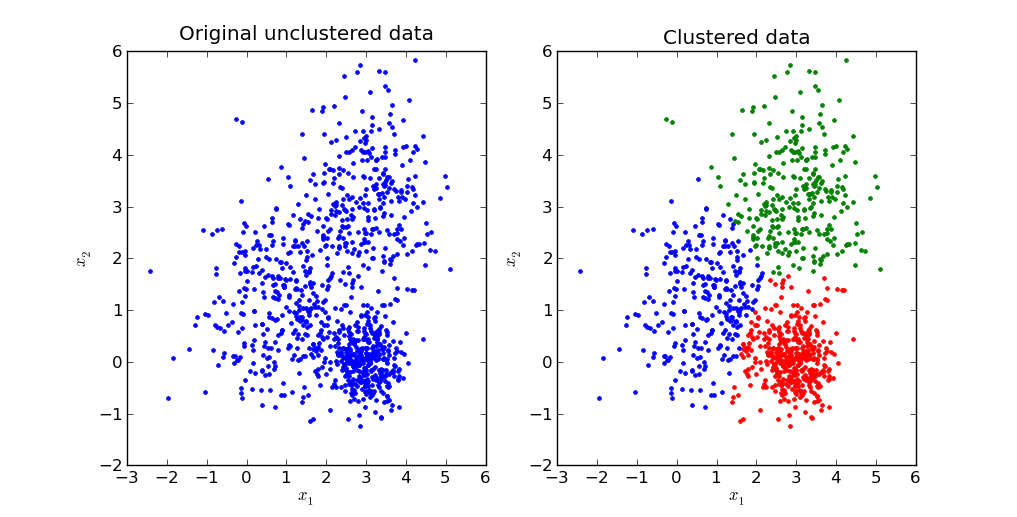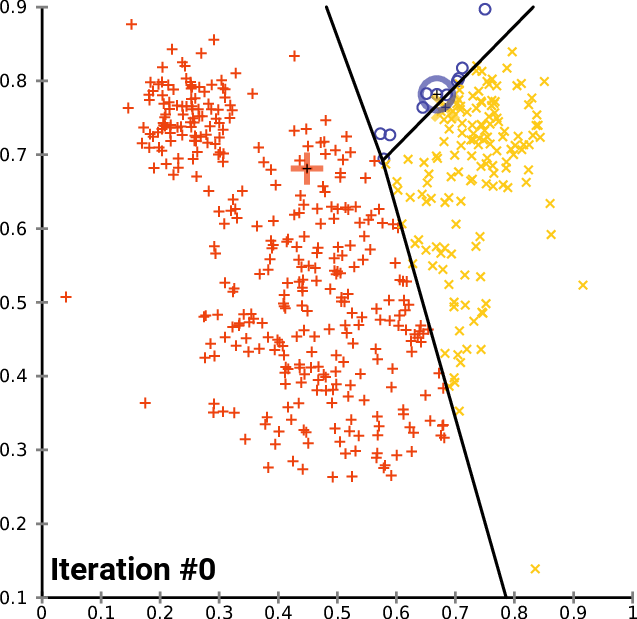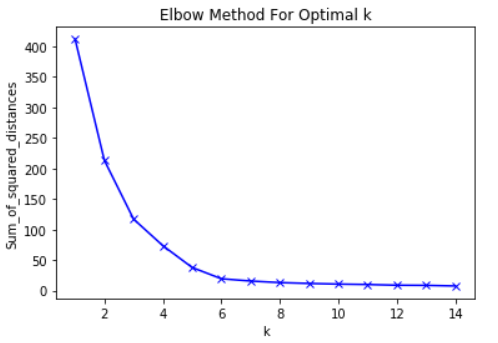# Introduction to K Means Clustering

Hey - Nick here! This page is a free excerpt from my new eBook Pragmatic Machine Learning, which teaches you real-world machine learning techniques by guiding you through 9 projects.

So far in this course, we have exclusively focused on supervised machine learning techniques.

This means that the data sets we have been working with have had input-output pairs. As an example, we worked with a breast cancer tumor data set where the input data was tumor characteristics and the output data was whether the tumor was malignant or benign.

This tutorial will introduce you to your first unsupervised machine learning model: K means clustering.

More specifically, this tutorial will provide a theoretical introduction to K means clustering before we learn to code K means clustering models in Python later.

## What is K Means Clustering?

K means clustering is an unsupervised machine learning algorithm.

This means that it takes in unlabelled data and will attempt to group similar clusters of observations together within your data.

K means clustering algorithms are highly useful for solving real-world problems. Here are a few use cases for this machine learning model:

• Customer segmentation for marketing teams
• Document classification
• Delivery route optimization for companies like Amazon, UPS, or FedEx
• Identifying and reacting to crime centers within a city
• Professional sport analytics
• Predicting and preventing cybercrime

The primary goal of a K means clustering algorithm is to divide a data set into distinct groups such that the observations within each group are similar to each other.

Here is a visual representation of what this looks like in practice:We will explore the mathematics behind a K means clustering in the next section of this tutorial.

## How Do K Means Clustering Algorithms Work?

The first step in running a K means clustering algorithm is to select the number of clusters. This number of clusters is the `K` value that is referenced in the algorithm's name.

Choosing the `K` value within a K means clustering algorithm is an important choice. We will talk more about how to chose a proper value of `K` later in this course.

Next, you must randomly assign each point in your data set to a random cluster. This gives our initial assignment which you then run the following iteration on until the clusters stop changing:

• Compute each cluster's centroid by taking the mean vector of points within that cluster
• Re-assign each data point to the cluster that has the closest centroid

Here is an animation of how this works in practice for a K means clustering algorithm with a `K` value of `3`. You can see the centroid of each of cluster represented by a black `+` character.As you can see, this iteration continues until the clusters stop changing - meaning data points are no longer being assigned to new clusters.

## Choosing A Proper `K` Value For K Means Clustering Algorithms

Choosing a proper `K` value for a K means clustering algorithm is actually quite difficult. There is no "right" answer for choosing the "best" `K` value.

One method that machine learning practitioners often use is called the elbow method.

To use the elbow method, the first thing you need to do is compute the sum of squared errors (SSE) for you K means clustering algorithm for a group of `K` values. SSE in a K means clustering algorithm is defined as the sum of the squared distance between each data point in a cluster and that cluster's centroid.

As an example of this step, you might compute the SSE for `K` values of `2`, `4`, `6`, `8`, and `10`.

Next, you will want to generate a plot of the SSE against these different `K` values. You will see that the error decreases as the `K` value increases.

This makes sense - the more categories you create within a data set, the more likely it is that each data point is close to the center of its specific cluster.

With that said, the idea behind the elbow method is to choose a value of `K` at which the SSE slows its rate of decline abruptly. This abrupt decrease produces an `elbow` in the graph.

As an example, here is a graph of SSE against `K`. In this case, the elbow method would suggest using a `K` value of approximately `6`.Importantly, `6` is just an estimate for a good value of `K` to use. There is never a "best" `K` value in a K means clustering algorithm. As with many things in the field of machine learning, this is a highly situation-dependent decision.

## Final Thoughts

In this tutorial, you had your first theoretical introduction to the K means clustering algorithm for unsupervised machine learning.

Here is a brief summary of what you learned in this article:

• Examples of unsupervised machine learning problems that the K means clustering algorithm is capable of solving
• The basic principles of what a K means clustering algorithm is
• How the K means clustering algorithm works
• How to use the elbow method to select an appropriate value of `K` in a K means clustering model